Next: Discrete Labels Mixture Model Up: tr04mw1 Previous: Paper Summary

# Modelling

We consider a mixture model on a regular spatial lattice of observations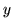, where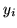is the observation at spatial location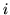, and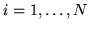. There are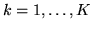distributions/classes in the mixture (e.g. for the FMRI data in section 6 we use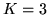classes, one for activation, a second for de-activation and a third for non-activation). The parameters of the class distributions are represented by the vector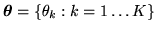.

We start by describing discrete classification mixture models. We will then go on to describe how we can approximate these models using the continuous weights mixture model, where we have replaced the discrete labels with vectors of continuous weights. We shall then see how the continuous weights spatial mixture model allows for the adaptive determination of the amount of spatial regularisation.

Subsections